## Example Questions

### Example Question #41 : Algebraic Fractions

Which of the following are answers to the equation below?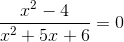1.2.3.1 only

3 only

1, 2, and 3

2 only

2 and 3

3 only

Explanation:

Given a fractional algebraic equation with variables in the numerator and denominator of one side and the other side equal to zero, we rely on a simple concept.  Zero divided by anything equals zero. That means we can focus in on what values make the numerator (the top part of the fraction) zero, or in other words,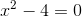The expression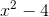is a difference of squares that can be factored as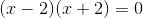Solving this forgives eitheror.  That means either of these values will make our numerator equal zero.  We might be tempted to conclude that both are valid answers.  However, our statement earlier that zero divided by anything is zero has one caveat. We can never divide by zero itself.  That means that any values that make our denominator zero must be rejected.  Therefore we must also look at the denominator.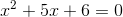The left side factors as follows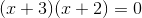This means that ifisor, we end up dividing by zero.  That means thatcannot be a valid solution, leavingas the only valid answer.  Therefore only #3 is correct.

### Example Question #1 : How To Find Excluded Values

Solve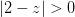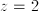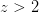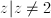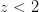No solutionsExplanation:

The absolute value will always be positive or 0, therefore all values of z will create a true statement as long as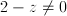. Thus all values except for 2 will work.

### Example Question #31 : Arithmetic Mean

If the average (arithmetic mean) of,, andis, what is the average of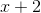,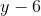, and?There is not enough information to determine the answer.Explanation:

If we can find the sum of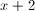,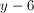, and 10, we can determine their average. There is not enough information to solve foror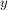individually, but we can find their sum,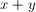Write out the average formula for the original three quantities.  Remember, adding together and dividing by the number of quantities gives the average: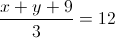Isolate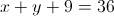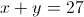Write out the average formula for the new three quantities: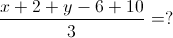Combine the integers in the numerator: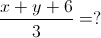Replacewith 27: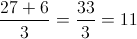### Example Question #1 : How To Find Excluded Values

Find the excluded values of the following algebraic fraction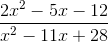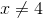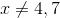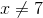The numerator cancels all the binomials in the denomniator so ther are no excluded values.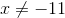Explanation:

To find the excluded values of a algebraic fraction you need to find when the denominator is zero. To find when the denominator is zero you need to factor it. This denominator factors into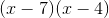so this is zero when x=4,7 so our answer is### Example Question #5 : How To Find Excluded Values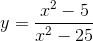For what value(s) of x is the function undefined?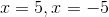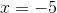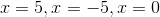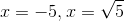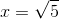Explanation:

When the denominator of a function is equal to 0, the function is undefined at that point. We can set x2-25 equal to 0 in order to find out what values of x make that true.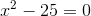We can factor to solve for x.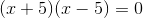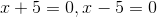is an incorrect answer because for this value of x, the function equals zero, but it is not undefined.

### Example Question #1 : How To Find Excluded Values

Find the extraneous solution for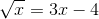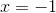There are no extraneous solutions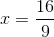Explanation: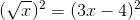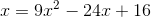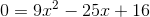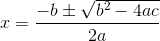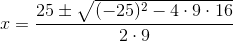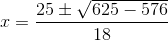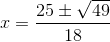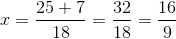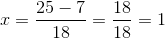Now lets plug these values into our original equation.

For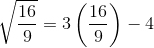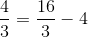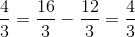So this isn't an extraneous solution.

For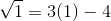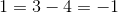Since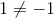is an extraneous solution.

### All SAT Math Resources